Courses

# Newton's Laws Of Motion MCQ Level-1

## 20 Questions MCQ Test | Newton's Laws Of Motion MCQ Level-1

Description
This mock test of Newton's Laws Of Motion MCQ Level-1 for Class 11 helps you for every Class 11 entrance exam. This contains 20 Multiple Choice Questions for Class 11 Newton's Laws Of Motion MCQ Level-1 (mcq) to study with solutions a complete question bank. The solved questions answers in this Newton's Laws Of Motion MCQ Level-1 quiz give you a good mix of easy questions and tough questions. Class 11 students definitely take this Newton's Laws Of Motion MCQ Level-1 exercise for a better result in the exam. You can find other Newton's Laws Of Motion MCQ Level-1 extra questions, long questions & short questions for Class 11 on EduRev as well by searching above.
QUESTION: 1

Solution:
QUESTION: 2

Solution:
QUESTION: 3

### Three blocks A, B and C are supended as shown in thefigure. Mass of each of blocks A and B in m. If systemis in equilibrium, and mass of C is M, then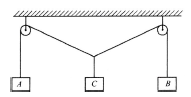Solution:
QUESTION: 4

A particle of small mass m is joined to very heavy bodyby a light string passing over a light pulley. Both bodiesare free to move. The total downward force on the pulley is

Solution:
QUESTION: 5

An object is suspended from a spring balance in a lift.The reading is 240 N when the lift is at rest. If the springbalance reading now changes to 220 N, then the lift ismoving

Solution:
QUESTION: 6

In the following arrangement, the system is initially atrest, The 5 kg block is now released. Assuming the pulleys and string to be massless and smooth, the acceleration of block C will be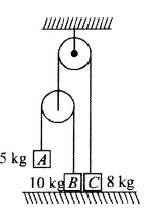Solution:
QUESTION: 7

As shown in the figure, if acceleration of M with respectto ground is 2 ms-2, then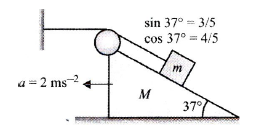Solution:
QUESTION: 8

A block A has a velocity of 0.6 ms-1 to the right,determine the velocity of cylinder B.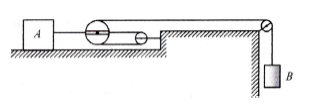Solution:
QUESTION: 9

For the pulley system shown, each of the cables at Aand b is given a velocity of 2 ms-1 in the direction of thearrow. Determine the upward velocity v of the load m.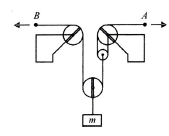Solution:
QUESTION: 10

A painter of mass M stands on a platform of mass mand pulls himself up by two ropes which hang over pulley as shown. He pulls each rope with force F and moves upward with a uniform acceleration a, Find a, neglecting the fact that no one could do this for long time.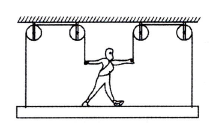Solution:
QUESTION: 11

A block is lying on the horizontal frictionless surfac.One end of a uniform rope is fixed to the block which ispulled in the horizontal direction by applying a force Fat the other end. If the mass of the rope is half themass of the block, the tension in the middle of the ropewill be

Solution:
QUESTION: 12

A 60 kg man stands on a spring scale in a lift. At someinstant, he finds that the scale reading has changedfrom 60 kg to 50 kg for a while and then comes back tooriginal mark. What should be concluded?

Solution:
QUESTION: 13

A monkey of mass 40 kg climbs on a massless rope ofbreaking strength 600 N. The rope will break if themonkey.

Solution:
QUESTION: 14

Three light strings are connected at the point P. A weight W is suspended from one of the string. End A of string AP and end B of string PB are fixed as shown. Inequilibrium, PB is horizontal and PA makes an angle of 600 with the horizontal. If the tension in PB is 30 N,then the tension in PA and weight W are, respectively, given by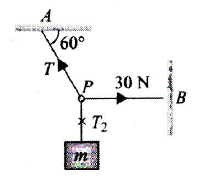Solution:
QUESTION: 15

Two particle A and B each of mass m, are kept stationaryby applying a horizontal force F = mg on particle B asshown in figure. then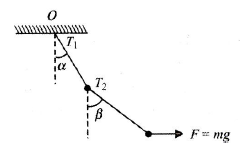Solution:
QUESTION: 16

A block of mass m is at rest with respect to a roughincline kept in elevator moving up with acceleration a.Which of following statements is correct?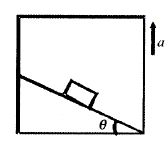Solution:
QUESTION: 17

In an arrangement shown below, the acceleration of block A and B are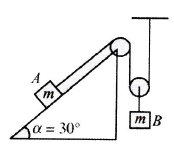Solution:
QUESTION: 18

A block of mass m1 lies on the top of fixed wedge as shown in Fig. (a) and another block of mass m2 lies on top of wedge which is free to move as shown in Fig (b). At time t = 0, both the blocks are released from rest from a vertical height h above the respective horizontal
surface on which the wedge is placed as shown. There is no friction between block and wedge in both the figures. Let T1 and T2 be the time taken by the blocks, respectively, to just reach the horizontal surface.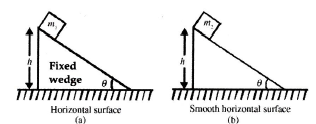Solution:
QUESTION: 19

In the figure shown, a person wants to rise a blocklying on the ground to a height h. In both the cases, if time required is same then in which case he has to exert more force. Assume pulleys and strings light.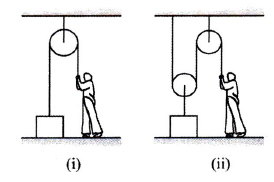Solution:
QUESTION: 20

In the shown arrangement below, if acceleration of B is aˆ , then find the acceleration of A.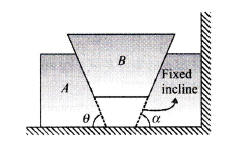Solution: